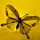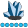whymath
Lapis Lazuli | Level 10

## Logic error of cumulative statement and lag function

This task is going to derive a time-series variable, The rule is:

X(t) = 1.5 * X(t-1) - 0.5 * X(t-2) + e;
X(1) = e;
X(2) = 1.5 * X(1) + e;

where e follows a standard normal distribution. Here is how I generate this sequence:

``````data have;
call streaminit(42);
do i=1 to 200;
e=rand("normal",0,1);
output;
end;
run;

data want;
set have;

*Method 1;
x+sum(0.5*x,-0.5*lag(x),e);

*Method 2;
y+0.5*y-0.5*lag(y)+e;

*Method 3;
retain z;
if _n_=1 then z=e;
if _n_=2 then z=1.5*z+e;

lag2_z=lag2(z);
if _n_>2 then z=1.5*z-0.5*lag2_z+e;
run;``````

Run this code, I just find only result of method 1 is right. Why Method 2 and Method 3 are wrong? How to fix them?

Thank you for any hint.

1 ACCEPTED SOLUTION

Accepted Solutions

## Re: Logic error of cumulative statement and lag function

With method 2, the problem is simply that the expression

``y+0.5*y-0.5*lag(y)+e;``

is to be understood as

``y+(0.5*y-0.5*lag(y)+e);``

which in the first iteration will set Y to 0 (the SUM statement, "Y+..." will initialize Y to 0, retain Y, and add whatever is after the plus sign, like

``````retain y 0;
y=sum(y,0.5*y-0.5*lag(y)+e);``````

But the second parameter evaluates to a missing value, meaning that Y stays 0 in the first iteration.

The method 1 calculation, on the other hand, is equivalent to

``````retain x 0;
x=sum(x,0.5*x,-0.5*lag(x),e);``````

which will set X to E on the first iteration.

In method 3, the problem is that you calculate Z before taking the LAG2 function the first two times, but after the LAG2 call the remaining times. Method 3 can be rewritten as e.g.

``````  retain z;

lag2_z=lag(z);

if _n_=1 then z=e;
else if _n_=2 then z=1.5*z+e;
else z=1.5*z-0.5*lag2_z+e;``````

Note that I changed from LAG2 to LAG, as the LAG call now always comes first.

2 REPLIES 2

## Re: Logic error of cumulative statement and lag function

With method 2, the problem is simply that the expression

``y+0.5*y-0.5*lag(y)+e;``

is to be understood as

``y+(0.5*y-0.5*lag(y)+e);``

which in the first iteration will set Y to 0 (the SUM statement, "Y+..." will initialize Y to 0, retain Y, and add whatever is after the plus sign, like

``````retain y 0;
y=sum(y,0.5*y-0.5*lag(y)+e);``````

But the second parameter evaluates to a missing value, meaning that Y stays 0 in the first iteration.

The method 1 calculation, on the other hand, is equivalent to

``````retain x 0;
x=sum(x,0.5*x,-0.5*lag(x),e);``````

which will set X to E on the first iteration.

In method 3, the problem is that you calculate Z before taking the LAG2 function the first two times, but after the LAG2 call the remaining times. Method 3 can be rewritten as e.g.

``````  retain z;

lag2_z=lag(z);

if _n_=1 then z=e;
else if _n_=2 then z=1.5*z+e;
else z=1.5*z-0.5*lag2_z+e;``````

Note that I changed from LAG2 to LAG, as the LAG call now always comes first.whymath
Lapis Lazuli | Level 10

## Re: Logic error of cumulative statement and lag function

Thank you very much, very detailed.
Discussion stats
• 2 replies
• 157 views
• 3 likes
• 2 in conversation